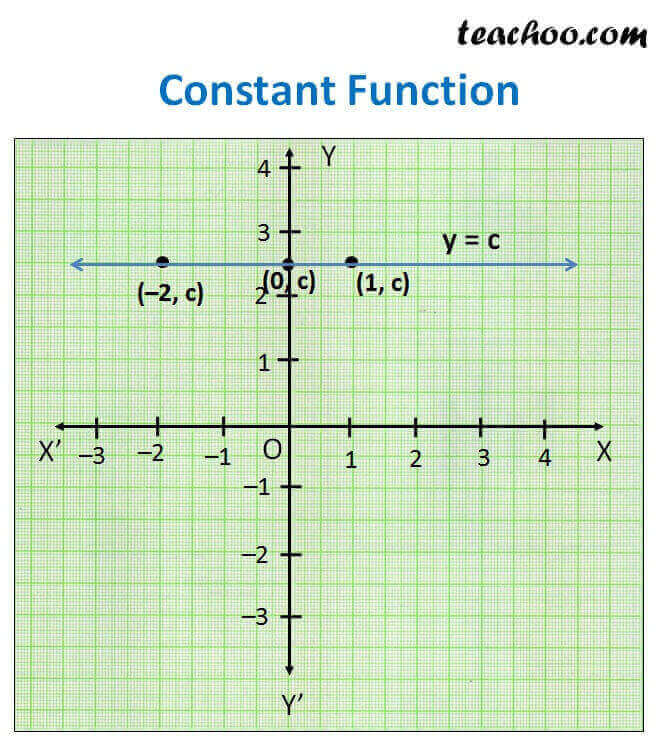Different Functions and their graphs

Chapter 2 Class 11 Relations and Functions
Concept wise

f: R R

f(x) = c for each x ∈ R

i.e. y = c for each x ∈ R

Here c is a constant.

Plotting graph

For all values of x, value of y will be c

 x 0 1 –2 y c c cHere,

Domain   = All values of x = R

Range = All values of y

= c (Since y = c)

So, Range = {c}

Learn in your speed, with individual attention - Teachoo Maths 1-on-1 Class